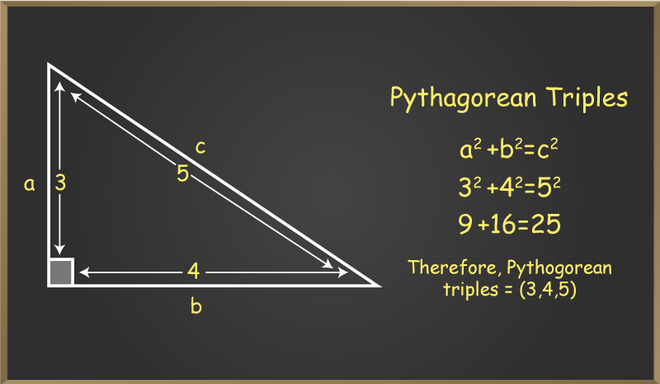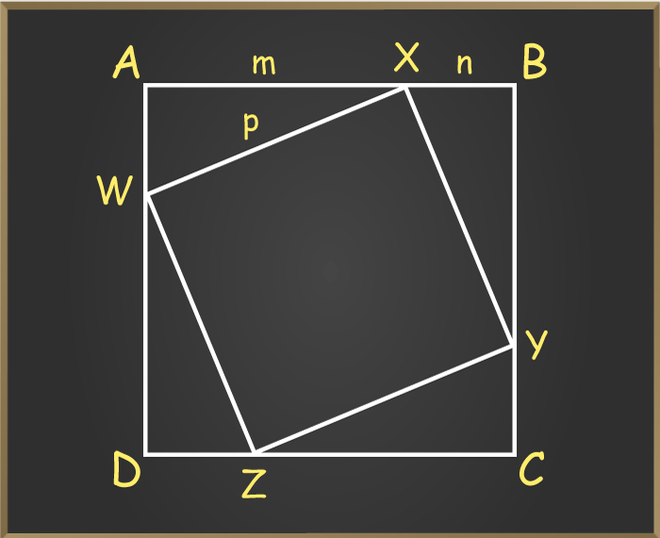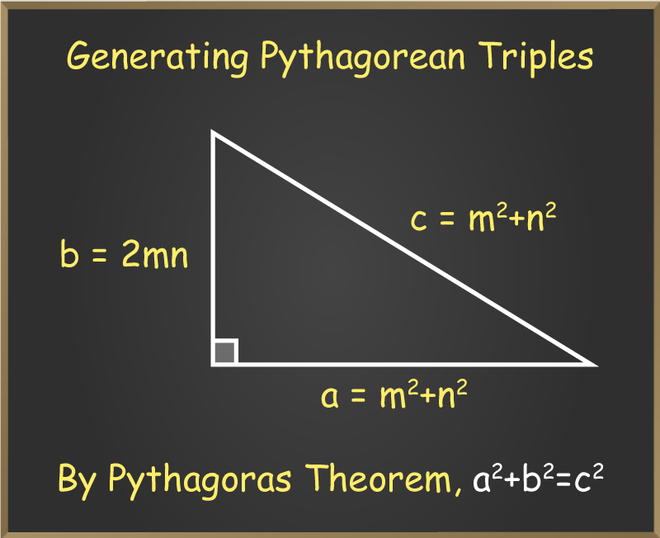Open in App
Not now

# Pythagorean Triples

• Difficulty Level : Expert
• Last Updated : 10 Jan, 2023

Pythagorean triples concept was used since ancient times by many greeks, Chinese and Indian philosophers. It is used to explain the relationship between the three sides (i.e., a, b, and c) of the right triangle. It states that in any right triangle, the square of the hypotenuse is equal to the sum of the square of the other two sides of the triangle. In this article, we will learn about the Pythagorean triples formula in detail, along with examples.

### What are Pythagorean Triples?

Pythagorean triples are used to find the three positive integers or terms that satisfy the Pythagorean theorem. Generally, these three terms can be written in the form (a, b, c), and the triangle formed by these terms is known as the Pythagorean triangle. Let us consider a right-angled triangle in which b is the base, a is the perpendicular, and c is the hypotenuse. So, according to the Pythagorean triples theorem: the sum of squares of any two sides is equal to the square of the third side.

a2 + b2 = c2

Here, a, b, and c are base, perpendicular, and hypotenuse.

## Examples of Pythagorean Triples

There is n number of Pythagorean triples. Take any Pythagoras triangle as an example. Let’s say the perpendicular of the triangle is 4 units, and the base is 3 units, then the hypotenuse will be:

a2 + b2 = c2

32 + 42 = c2

c2 = 9 + 16

c = √25 = 5 units.So, the Pythagorean triples are (3, 4, and 5). Many other Pythagorean triples can be generated with the help of these basic Pythagorean triples. The best way to obtain more triples is to scale them up, that is (3n, 4n, and 5n). Here, n is the positive integer value.

## Pythagorean Triples Formula

If the triangle given is a right-angled triangle, then the set of the sides of the triangle gives Pythagorean triples. Pythagorean triples are obtained from the Pythagoras theorem. The pythagoras theorem states that in a right-angled triangle, the square of the hypotenuse is equal to the sum of the squares of base and perpendicular. Let’s say the perpendicular is denoted by ‘a’, the base is denoted by ‘b’, and the hypotenuse is denoted by ‘c’, then the Pythagorean triples formula will be:

c2 = a2 + b2

## Pythagorean Triples Proof

We can prove the Pythagorean triples Formula in many ways, but here we use the algebraic method. In this method, we use the terms shown in the below figure.Step 1: We have four right-angled triangles with base m, perpendicular n, and hypotenuse p. Now arrange these triangles so that they make two squares one is outer square ABCD, whose side is m+n, and another one is inner square WZXY, whose side is c.

Step 2: Now we find the area of the inner, outer square, and triangles:

Area of outer square ABCD = (m + n)2

Area of inner square WXYZ = (p)2

Area of one triangle = 1/2(m × n)2

Area of four triangles = 4 × 1/2(m × n)2 = 2(m × n)2

Step 3: As we know that the area of square ABCD = Area of square WXYZ + Area of four triangles

So (m + n)2 =  2(m × n)2 + p2

m2 + 2 × m × n + n2 = 2 × m × n + p2

m2 + n2 = p2

Hence Pythagorean triples Formula is proved.

## How to Form Pythagorean Triples?

Pythagorean triples can be positive integers. We can say there are two cases that can be generated. The numbers can either be odd or even. Below are the cases explained in detail:

### If the Number is Odd

The below formula can be used to find the triples. If we have an odd number (1, 3, 5, 7, 9, etc.) that can be taken as m, then the two numbers can be found by putting m in the formula.

(m, (m2 – 1)/2 , (m2 + 1)/2)

Here m should be greater than 1.

Example: If m = 3, find the rest of the Pythagorean triples.

Solution:

(m2 -1)/2 = (32 – 1)/2 = 8/2 = 4.

(m2 + 1)/2 = (32 + 1)/2 = 10/2 = 5

Therefore, the Pythagorean triples are (2, 4, 5).

### If the Number is Even

The below formula can be used to find the triples. If we have an even number (2, 4, 6, 8,10, etc.) that can be taken as m, then the other two numbers can be found by putting m in the formula.

(m, (m2 – 4)/4, (m2 + 4)/4)

Here m should be greater than 2.

Example: Find the rest of the Pythagorean triples if m = 4.

Solution:

(m2 – 4)/4 = (44 – 4)/4 = 252/4 = 63.

(m2 + 4)/4 = (44 + 4)/4 = 260/4 = 230/2 = 115.

Therefore, the Pythagorean triples are (4, 63, 115).

Note: Even if the method helps solve and find infinitely many Pythagorean triples, it still cannot find them all. Fir instance, the Pythagorean triples (20, 21, 29) cannot be formed using this technique.

## How to Generate Pythagorean Triples?

In order to generate Pythagorean triples, the Pythagorean triplet checker is used. Pythagorean triplet checker is the formula that is defined specifically to find out the values of Pythagorean triples. Assume the sides of the right-angled triangle are a, b and c. Now, m and n are the two integers that will be used in order to find the values of a, b, and c.

• a, b, and c are the sides of the right-angled triangle.
• m and n are the co-prime numbers that are positive numbers. Here, m>n.In the above figure, it can be easily seen that the values of a, b and c are dependent upon m and n. The sides in terms of m and n are defined as:

• a is the perpendicular of the triangle here, and a = 2mn.
• b is the base of the triangle, and b = m2 – n2.
• c is the hypotenuse of the triangle, and c = m2 + n2.

Now simply assume co-prime natural numbers in order to find the values of Pythagorean triples. It is important to note that m must be greater than n.

Example: Find the Pythagorean triples when the values of m and n are 3 and 2, respectively.

Solution:

Pythagorean triples checker is,

• a = 2mn
• b = m2 – n2
• c = m2 + n2

Therefore, putting m = 3 and n = 2.

a = 2 × 3 × 2 = 12 units.

b = 32 – 22 = 9 – 4 = 5 units

c = 33 + 22 = 9 + 4 = 13 units.

Therefore, the Pythagorean triples are (12, 5, 13).

## List of Pythagorean Triples

Below is the Pythagorean triples list where the value of c is greater than 100:

These values verify the formula a2 + b2 = c2.

## Types of Pythagorean Triples

### Primitive Pythagorean Triples

Primitive Pythagoras triples are also known as Reduced triples. The greatest common factor of these triples are 1. Or we can say that primitive Pythagorean triples are those triples in which the three numbers do not have any common divisor other than one. Such type of triples only contains one even positive number among the three given three numbers.

Example: 3, 4, 5

3, 4, 5 are Pythagorean triples as they satisfy the Pythagorean triples formula also the greatest common factor of 3, 4, 5 is 1.

### Non-Primitive Pythagorean Triples

Non-primitive Pythagoras triples are also known as imprimitive Pythagorean triples. Non-primitive Pythagorean triples are those triples in which the three numbers have a common divisor. Such types of triples can contain more than one even positive number among the three given three numbers.

Example: 6, 8, 10

6, 8, 10 are Pythagorean triples as they satisfy the Pythagorean triples formula but the greatest common factor of 6, 8, 10 is not equal to 1 so these are non – primitive Pythagorean triples.

## Properties of Pythagorean Triples

• Pythagorean triples are the sides of a right-angled triangle, represented as m, n, p.
• These numbers satisfy the Pythagorean triples formula m2 + n2 = p2.
• The sides m and n are the sides of a right triangle, which represent perpendicular and base, while p is the hypotenuse.
• Pythagorean Triplet consists of all even numbers, or two odd numbers and one even number.
• All three numbers of a Pythagorean Triplet can never be odd.

## Solved Examples on Pythagoras Triples

Example 1: Find Pythagorean triples if m = 8.

Solution:

This is the case when the number is even:

Given m = 8,

So, (m2 – 4)/4 = (64 – 4)/4 = 15

(m2 + 4)/4 = (64 + 4)/4 = 17

Hence Pythagorean triples are 8, 15, 17.

Example 2: Find Pythagorean triples if m = 9.

Solution:

This is the case when the number is odd:

Given m = 9,

So, (m2 – 1)/2 = (81 – 1)/2 = 40

(m2 + 1)/2 = (81 + 1)/2 = 41

Hence Pythagorean triples are 9, 40, 41.

Example 3: Find Pythagorean triples, one of whose members is 13.

Solution:

Take m = 13,

So, (m2 – 1)/2 = (169 – 1)/2 = 84

(m2 + 1)/2 =(169 + 1)/2 = 85

Hence Pythagorean triples are 13, 84, 85.

Example 4: Checking if (6, 8, 10) is a Pythagorean triplet or not.

Solution:

Let us take m = 6, n = 8, and p = 10

According to the formula

m2 + n2 = p2

We get

(6)2 + (8)2 = (10)2

36 + 64 = 100

100 = 100

Here L.H.S = R.H.S

Hence proved that (6, 8, 10) is a Pythagorean triplet.

Example 5: If (y, 84, 85) is a Pythagorean triplet, then find the value of y.

Solution:

Let us take m = y, n = 84, and p = 85

According to the formula

m2 + n2 = p2

We get

(y)2 + (84)2 = (85)2

y2 + 7056 = 7225

y2 = 169

y = 13

## FAQs on Pythagorean Triples

Question 1: What are Pythagorean triples?

Pythagorean triples are the natural numbers that satisfy the pythagoras theorem. Therefore, the Pythagorean triples satisfy the formula c2 = a2 + b2, here, c is the hypotenuse of the right-angled triangle, a and b are the legs of the triangle.

Question 2: What are the five most common Pythagorean triples?

The five most common Pythagorean triples are: (3, 4, 5), (5, 12, 13), (6, 8, 10), (9, 12, 15), (15, 20, 25).

Question 3: How to find Pythagorean triples?

The Pythagorean triples can be easily obtained by the help of pythagoras theorem, if two triples are already given, the third triplet can be found:

a2 + b2 = c2

Where, a and b are the legs of the triangle and c is the hypotenuse of the triangle.

There are times when only one Pythagorean triplet is given, two cases arise in this situation, if the value of m given is odd, the  formula used for the Pythagorean triples are (m, (m2 – 1)/2, (m2 + 1)/2). If the value of m given is even, the  formula becomes (m, (m2 – 4)/4, (m2 + 4)/4).

Question 4: Can Pythagorean triples be decimals?

Pythagorean triples are the positive integers, that is, natural numbers that satisfy the pythagoras theorem, Therefore, they can not be in decimal form.

Question 5: What is the scaling of triples?